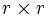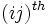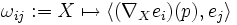# Matrix of connection forms

## Definition

Suppose$M$ is a differential manifold and$\nabla$ is a connection on$M$. The matrix of connection forms at a point$p \in M$ is a way of describing the connection in terms of coordinate charts. The matrix of connection forms is dependent on the choice of coordinate chart. It is described as follows.

Suppose$U$ is an open set at$p$ such that the bundle$E$, restricted to$U$, is trivial. Suppose, further, that$e_1,e_2,\ldots,e_r$ is the collection of constant vector fields for basis directions in$U$. Then the matrix of connection forms at$p$ is given by a$r \times r$ matrix where the entry in the$(ij)^{th}$ position is the 1-form:$\omega_{ij} := X \mapsto \left \langle (\nabla_X e_i)(p), e_j \right \rangle$

In other words, the 1-forms are chosen so that:$\nabla_Xe_i = \sum_j \omega_{ij}(X)(p) e_j$

## Relation with Christoffel symbols

The matrix of connection forms is closely related to the Christoffel symbols. To obtain the Christoffel symbols from the matrix of connection forms, we need to choose a basis for the tangent space at the point, and express the 1-form in terms of the coefficients of its dual basis.

The key difference is thus:

• The matrix of connection forms does not depend on a choice of basis for$TM$. It only depends on a local trivialization for the bundle$E$.
• The Christoffel symbols, on the other hand, depend on a choice of basis for$TM$ as well.